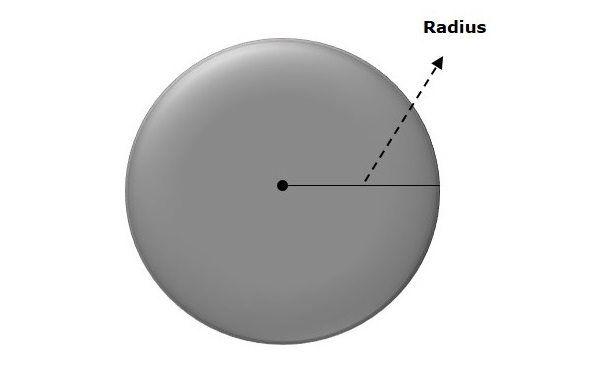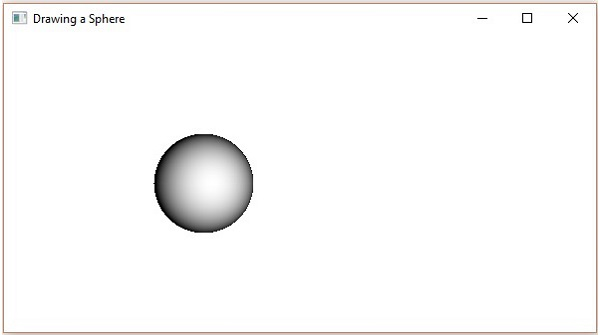# JavaFX - 3D Shape Sphere

A sphere is a perfectly round geometrical object in a three-dimensional space that is the surface of a completely round shaped ball.

A sphere is defined as the set of points that are all at the same distance r from a given point in a 3D space. This distance r is the radius of the sphere and the given point is the centre of the sphere.In JavaFX, a sphere is represented by a class named Sphere. This class belongs to the package javafx.scene.shape. By instantiating this class, you can create a sphere node in JavaFX.

This class has a property named radiusof double datatype. It represents the radius of a Sphere. To draw a Sphere, you need to set values to this property by passing it to the constructor of this class at the time of instantiation as follows −

```Sphere sphere = new Sphere(radius);
```

Or, by using a method named setRadius() as follows −

```setRadius(value);
```

## Steps to Draw 3D Sphere

Follow the steps given below to Draw a Sphere (3D) in JavaFX.

### Step 1: Creating a Class

Create a Java class and inherit the Application class of the package javafx.application and implement the start() method of this class as follows.

```public class ClassName extends Application {
@Override
public void start(Stage primaryStage) throws Exception {
}
}
```

### Step 2: Creating a Sphere

You can create a Sphere in JavaFX by instantiating the class named Sphere, which belongs to a package javafx.scene.shape. You can instantiate this class as follows.

```//Creating an object of the class Sphere
Sphere sphere = new Sphere();
```

### Step 3: Setting Properties to the Sphere

Set the radius of the Sphere using the method named setRadius() as shown below.

```//Setting the radius of the Sphere
```

### Step 4: Creating a Group Object

In the start() method, create a group object by instantiating the class named Group, which belongs to the package javafx.scene.

Pass the Sphere (node) object, created in the previous step, as a parameter to the constructor of the Group class. This should be done in order to add it to the group as shown below −

```Group root = new Group(sphere);
```

### Step 5: Creating a Scene Object

Create a Scene by instantiating the class named Scene, which belongs to the package javafx.scene. To this class, pass the Group object (root) created in the previous step.

In addition to the root object, you can also pass two double parameters representing height and width of the screen along with the object of the Group class as follows.

```Scene scene = new Scene(group ,600, 300);
```

### Step 6: Setting the Title of the Stage

You can set the title to the stage using the setTitle() method of the Stage class. The primaryStage is a Stage object, which is passed to the start method of the scene class as a parameter.

Using the primaryStage object, set the title of the scene as Sample Application as follows.

```primaryStage.setTitle("Sample Application");
```

### Step 7: Adding Scene to the Stage

You can add a Scene object to the stage using the method setScene() of the class named Stage. Add the Scene object prepared in the previous steps using this method as shown below.

```primaryStage.setScene(scene);
```

### Step 8: Displaying the Contents of the Stage

Display the contents of the scene using the method named show() of the Stage class as follows.

```primaryStage.show();
```

### Step 9: Launching the Application

Launch the JavaFX application by calling the static method launch() of the Application class from the main method as shown below.

```public static void main(String args[]){
launch(args);
}
```

### Example

The following program shows how to generate a Sphere using JavaFX. Save this code in a file with the name SphereExample.java.

```import javafx.application.Application;
import javafx.scene.Group;
import javafx.scene.Scene;
import javafx.stage.Stage;
import javafx.scene.shape.Sphere;

public class SphereExample extends Application {
@Override
public void start(Stage stage) {
//Drawing a Sphere
Sphere sphere = new Sphere();

//Setting the properties of the Sphere

sphere.setTranslateX(200);
sphere.setTranslateY(150);

//Creating a Group object
Group root = new Group(sphere);

//Creating a scene object
Scene scene = new Scene(root, 600, 300);

//Setting title to the Stage
stage.setTitle("Drawing a Sphere - draw fill");

stage.setScene(scene);

//Displaying the contents of the stage
stage.show();
}
public static void main(String args[]){
launch(args);
}
}
```

Compile and execute the saved java file from the command prompt using the following commands.

```javac SphereExample.java
java SphereExample
```

On executing, the above program generates a JavaFX window displaying a Sphere as shown below.javafx_3d_shapes.htm